Lecture 25: Mazes and States

Last Time

• Considered solving mazes
• Developed depth-first strategy
• Saw depth-first can yield inefficient solutions

Another Strategy

Explore in all directions simultaneously!

• Consider all possible first steps
• Then all possible second steps
• ignore previously visited cells

• Explore all possible next steps

Illustration: Depth 1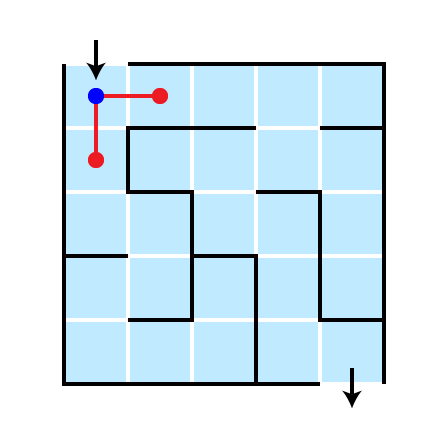Illustration: Depth 2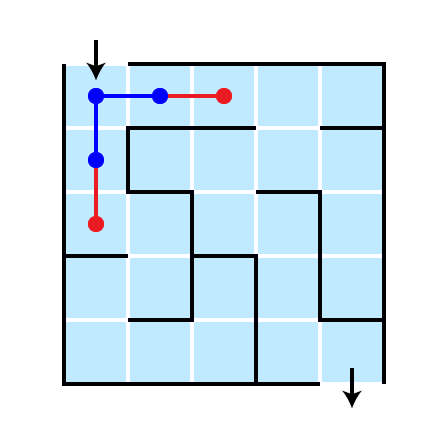Illustration: Depth 3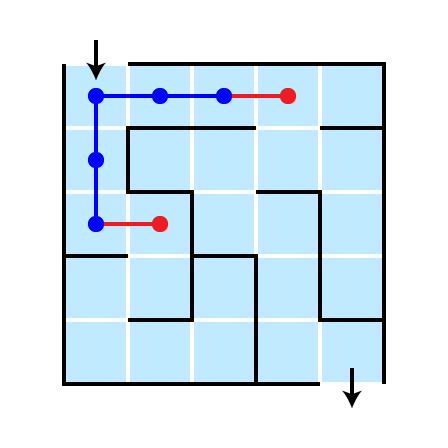Illustration: Depth 4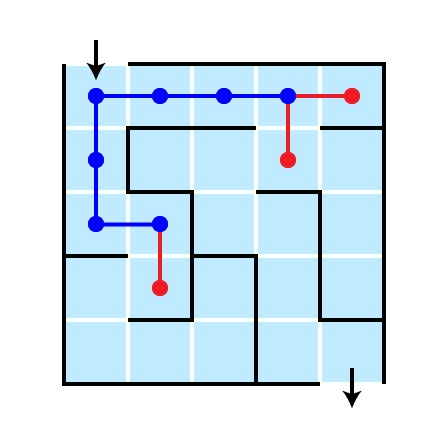Return Solution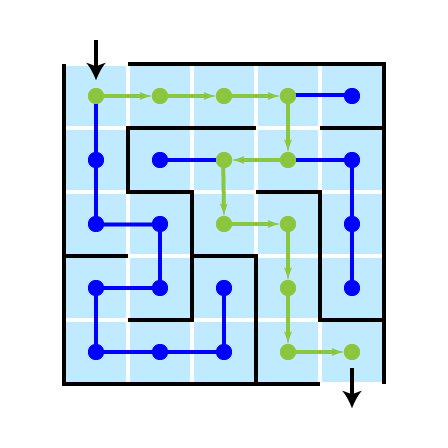A Nice Feature

The shortest path from start to goal is found

• why?

Implementation Notes

• again, store visited and active cells
• active cells are boundary between visited and unvisited
• store active cells in a queue
• all cells at distance $d$ from start are visited before any cell at distance $d+1$ is visited
• initially, active and visited are just starting cell
• each cell stores its parent cell
• parent is cell from which cell was visited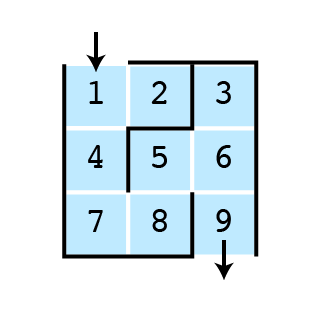Two Solution Philosophies

1. Depth-first
• Keep going until you can’t go any farther, then backtrack
• Naturally suited to stack ADT
• Naturally suited to recursive solution
• Exhaustively search all cells in increasing distance from start
• Keep track of “parent” cell for each cell

Features of Mazes

1. Comprised of cells: states
2. Cells having neighboring cells
• can move from one cell to its neighbors
• moving from one state to another is a transition
3. Have starting position
4. Have goal position(s)

Objective: find a sequence of transitions from initial state to gaol state

Two Stratgies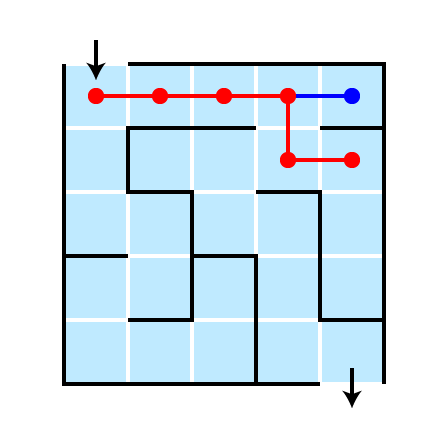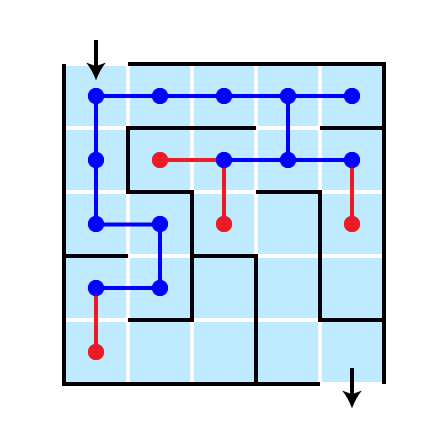More Problems, Same Features

• Driving directions
• Solving puzzles
• Playing games
• tic-tac-toe
• chess

Generic Problem

Input:

• Initial state
• Transition rules
• Goal state(s)

Output:

• Sequence of transitions from initial state to goal state

Example: Tower of Hanoi

• 3 pegs
• $n$ disks sit atop pegs
• move one peg at a time
• cannot place larger disk atop smaller

Initial State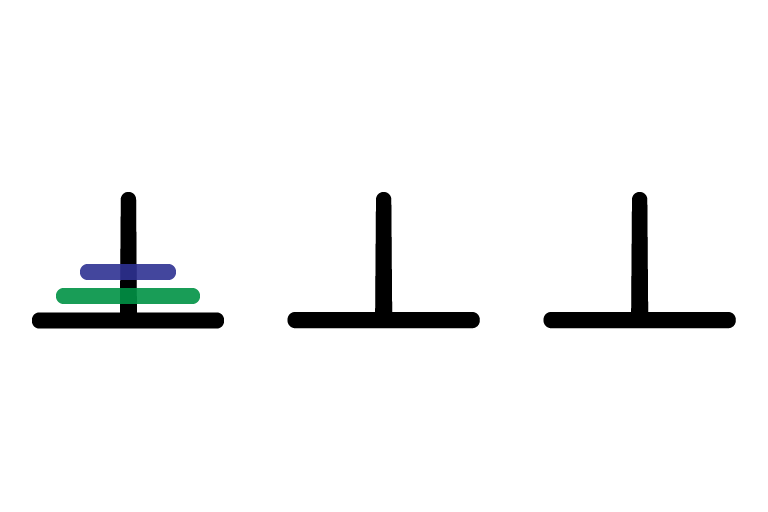Goal State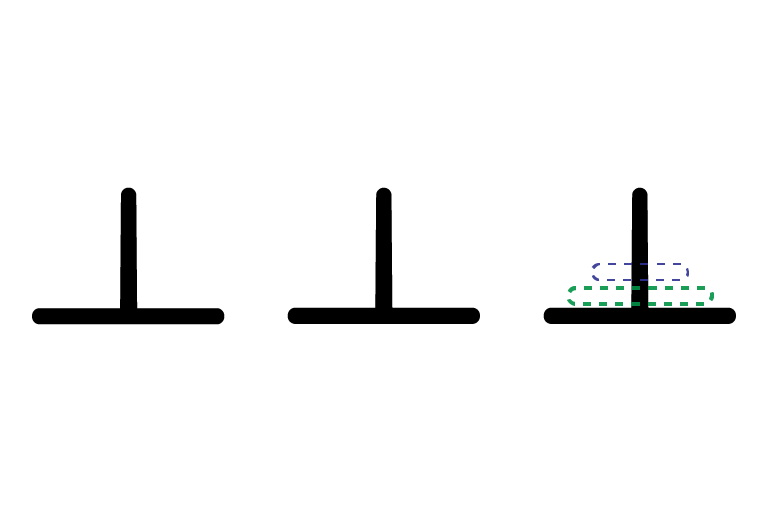Transitions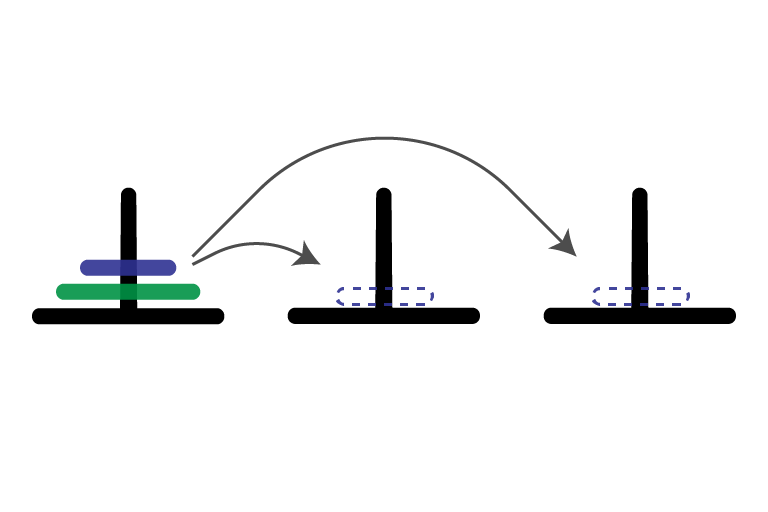States and Transitions

• states are the configurations of the game
• collection of disks on each peg
• transitions connect one state to another if a single legal move transforms one to the other

State Diagram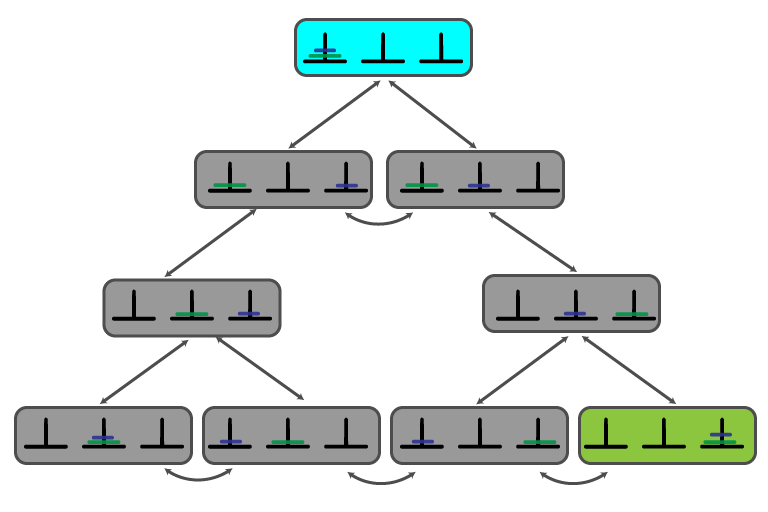Solving ToH

• can use same strategy as maze solutions
• start at start state (blue above)
• search entire state space for goal state (green above)
• use DFS or BFS
• return path from start to goal state

What to Implement?

• class representing states
• method for computing neighbors of a state
• method for determining if a state is a goal

Abstract State Representation

abstract class State {
private State parent = null;
public abstract ArrayList<State> getNeighbors ();
public State getParent() { return parent; }
public void setParent(State parent) { this.parent = parent; }
public abstract boolean isGoal ();
}

Abstract Solution

• Use same strategies as maze
• depth-first search (DFS)
• Same idea can be applied to any problem that can be encoded as:
• states and transitions
• given initial state
• want to find goal state
• Can write generic solver program
• solves problems without referencing which problem is being solved!

Generic DFS Solution

private static boolean getDFSolution (Stack<State> active, ArrayList<State> visited) {

if (active.peek().isGoal())
return true;

State cur = active.peek();
ArrayList<State> neighbors = cur.getNeighbors();

for (State s : neighbors) {
if (!visited.contains(s)) {
active.push(s);

if (getDFSolution(active, visited))
return true;
}
}

active.pop();
return false;
}

Generic BFS Solution

private static State getBFSolution (State start) {
ArrayList<State> visited = new ArrayList<State>();
Queue<State> active = new Queue<State>();

State next = start;

while (!next.isGoal()) {
for (State s : next.getNeighbors()) {
if (!visited.contains(s)) {
active.enqueue(s);
s.setParent(next);
}
}
if (!active.isEmpty())
next = active.dequeue();
else
return null;
}
return next;
}

How did we get here?

• represent input/states as interacting objects: object oriented design
• solve problems generically (polymorphism, interfaces, generic classes)
• same code can be used to solve many different problems
• store/access/manipulate states in data structures (arrays, linked lists)
• implement abstract data types (stacks, queues)
• generate solution to problems recursively (DFS)

Next Semester

COSC 211: Data Structures

• Deep dive into how to effectively store, organize, access, and manipulate data